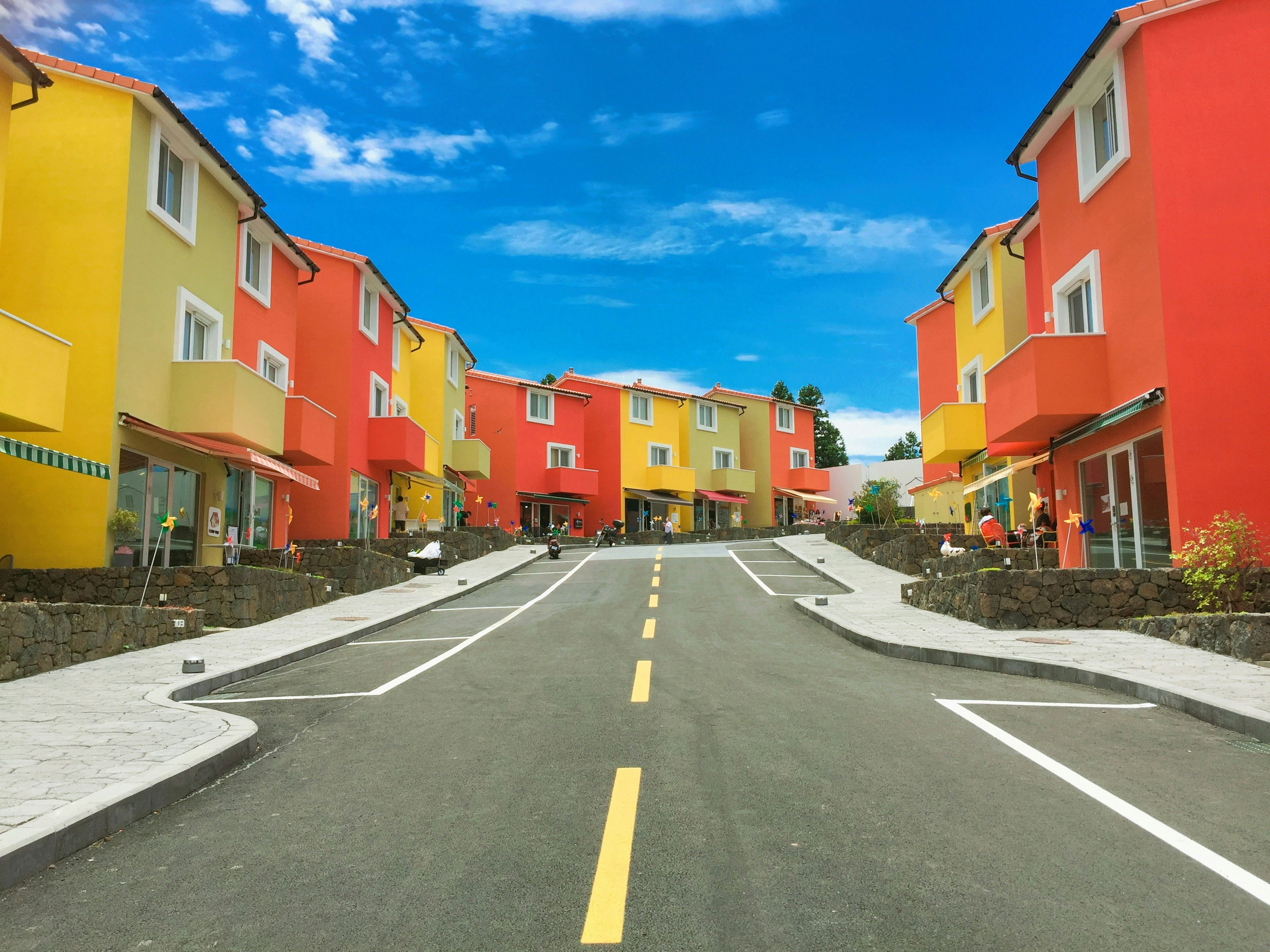Akhil Chaudhary
NIT Warangal graduate, Software Engineer at Wipro.

# K Nearest NeighborsThe k-nearest neighbors (k-NN) is a non-parametric, supervised learning algorithm that can be used to solve both regression and classification problems. The logic behind k-NN is that similar data points exist in close proximity to each other.

In classification problems, k-NN assigns the data point a class belonging to the majority of its k-nearest neighbors. In regression problems, k-NN predicts the output as the average of values of the k-nearest neighbors.

The k-NN algorithm relies on distance measurement, so if the features have varying scales and ranges, normalizing the data improves the training accuracy dramatically.

Choosing the ideal value of k is the most important step in its implementation. This is done by iterating the model training over different k values starting from 1.

At k = 1, the model is usually unstable, as it only outputs the state of its nearest neighbors, i.e. predictions are random. As k goes on increasing, averaging and majority voting improve the model accuracy dramatically up to a certain point. Once the error starts increasing again, this is the point when it is clear that we’ve pushed the value of k too far.

The k-NN algorithm is easy to implement, needs no tuning or assumptions, and can be used in both regression and classification. One major drawback of the algorithm is that it gets significantly slower as the value of k increases. This makes it an impractical choice in cases where we need predictions rapidly or in real-time.

However, provided one has sufficient computing resources, k-NN is very useful in applications where solutions depend on the similarity of objects.

# k-NN CLASSIFIER CODE

from sklearn.neighbors import KNeighborsClassifier

nb = KNeighborsClassifier(n_neighbors=3)

nb.fit(data.iloc[:,0:4], data['Name'])

# Predicted class

print(nb.predict(test))

# 3 nearest neighbors

print(nb.kneighbors(test))